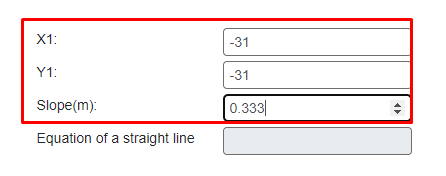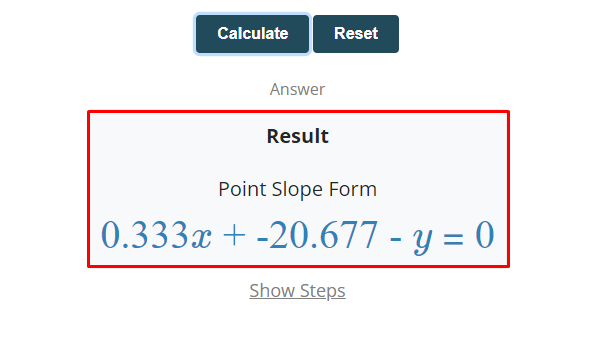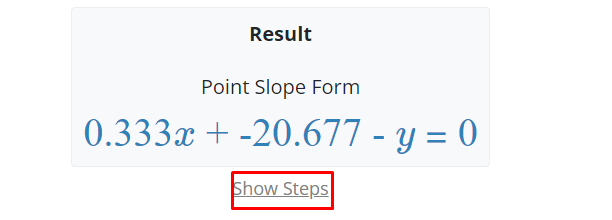# Introduction to Point-slope form with definition, formula, and examples

The point-slope form is widely used in algebra to find the equation of a straight line by using the slope and the points of the line. The linear equation of the straight line is usually used in various algebraic and differential equations.

In the equation of the straight line, the power of each variable is one so it is also known as the linear equation. In algebra, the equation of the straight line can be found by using different methods.

These methods are the point-slope form, slope-intercept form, and two-point intercept form. In this article, we will learn the definition, formula, and how to calculate the equation of a line by using the point-slope form along with a lot of examples.

## What is the point-slope form?

In algebra, a general method used to find the equation of the straight line or linear equation of the line is said to be the point-slope form. The slope of the line is very essential for finding the equation of the straight line using the point-slope form.

The slope is the steepness of the line. it can be measured by using the equation of the line or two points method. The slope can be positive, negative, zero, or undefined. The slope is the ratio of the rise and run of the points of the line.

The rise is the change in the points of y and the run is the change in the points of x. It can also be written in the form of a fraction such as a rise over a run. The formula for finding the slope of the line sing two points methods is given below.

The slope of the line = rise : run = rise / run

The slope of the line = Δy / Δx = y2 – y1 / x2 – x1

For solving the equation of the point-slope form, the slope and the points must be known. You can easily find the slope of the line by using the given points of the line. The equation of the point-slope form for finding the linear equation of the straight line is:

y – y1 = m (x – x1)

where x and y are the fixed variables of the equation of the straight line, x1 and y1 is the given pair of the line, and m is the slope of the line.

generally, the equation of the point-slope form is derived from the equation of the slope. It is mostly used to find the slope of the line if a pair of points of the line is given. The equation of the straight line can be calculated easily by using the equation of the point-slope form.

### How to calculate the point-slope form to find the linear equation?

The equation of the straight line can be found easily by using the general formula of the point-slope form. The slope of the line and a pair of points must be known for calculating the equation of the point-slope form.

If the slope of the line is not given, you have to calculate it. Follow the following steps to find the equation of the straight line by using the point-slope form method.

• Find the slope of the line, If the slope of the line is not given.
• Write the general formula of the equation of the line.
• Put any pair of points and the slope of the line.
• The result must be the equation of the straight line.

Following are a few solved examples of the point-slope form.

Example 1: For positive integers

Find the equation of the straight line using the point-slope form method, if the points are (11, 32), (23, 45)

Solution

Step 1: Take the given pair of point of the line.

x1 = 11, x2 = 23, y1 = 32, y2 = 45

Step 2: Take the general equation to find the slope of the line.

The slope of the line = Δy / Δx = y2 – y1 / x2 – x1

Step 3: Substitute the values of x and y in the formula of the slope.

The slope of the line = m = (y2 – y1) / (x2 – x1)

The slope of the line = m = (45 – 32) / (23 – 11)

The slope of the line = m = (13) / (12)

The slope of the line = m = 13 / 12

The slope of the line = m = 1.0834

Step 4: Now take the general equation of the point-slope form.

y – y1 = m (x – x1)

Step 5: Substitute the calculated slope and any pair of points in the above formula to fin the equation of the straight line.

y – y1 = m (x – x1)

y – (32) = 1.0834(x – 11)

y – 32 = 1.0834x – 11.9174

y – 32 – 1.0834x + 11.9174 = 0

y – 1.0834x – 20.0826 = 0

1.0834x – y + 20.0826 = 0

Example 2: For negative integers

Find the equation of the straight line using the point-slope form method, if the points are (-31, -31), (-43, -35)

Solution

Step 1: Take the given pair of point of the line.

x1 = -31, x2 = -43, y1 = -31, y2 = -35

Step 2: Take the general equation to find the slope of the line.

The slope of the line = Δy / Δx = y2 – y1 / x2 – x1

Step 3: Substitute the values of x and y in the formula of the slope.

The slope of the line = m = (y2 – y1) / (x2 – x1)

The slope of the line = m = (-35 – (-31)) / (-43 – (-31))

The slope of the line = m = (-35 + 31) / (-43 + 31)

The slope of the line = m = (-4) / (-12)

The slope of the line = m = (4) / (12)

The slope of the line = m = 1 / 3

The slope of the line = m = 0.333

Step 4: Now take the general equation of the point-slope form.

y – y1 = m (x – x1)

Step 5: Substitute the calculated slope and any pair of points in the above formula to fin the equation of the straight line.

y – y1 = m (x – x1)

y – (-31) = 0.333(x – (-31))

y + 31 = 0.333(x + 31)

y + 31 = 0.333x – 10.323

y + 31 – 0.333x – 10.323 = 0

y – 0.333x + 20.677 = 0

0.333x – y + 20.677 = 0

You can also use a point slope calculator to find the equation of the straight line in a fraction of seconds. Follow the below steps to find the equation of the straight line by using this tool.

Step 1: Input the values of x1, y1, & slope m in the required input boxes.Step 2: Click the calculate button to get the result.Step 3: The result will come in a fraction of seconds below the calculate button.Step 4: Press the show steps button to view the solution with steps.#### Summary

The point-slope form is a famous method to find the linear equation of the straight line. The slope of the line plays a vital role in this method. You can grab all the basics of the point-slope form by learning the above post.#### Editor

Editorial Team of Mera Xaam.